Call Blocker / Telemarketer Stopper

Stop Those Cold Calls Cold!

IntermediateFull instructions provided4,086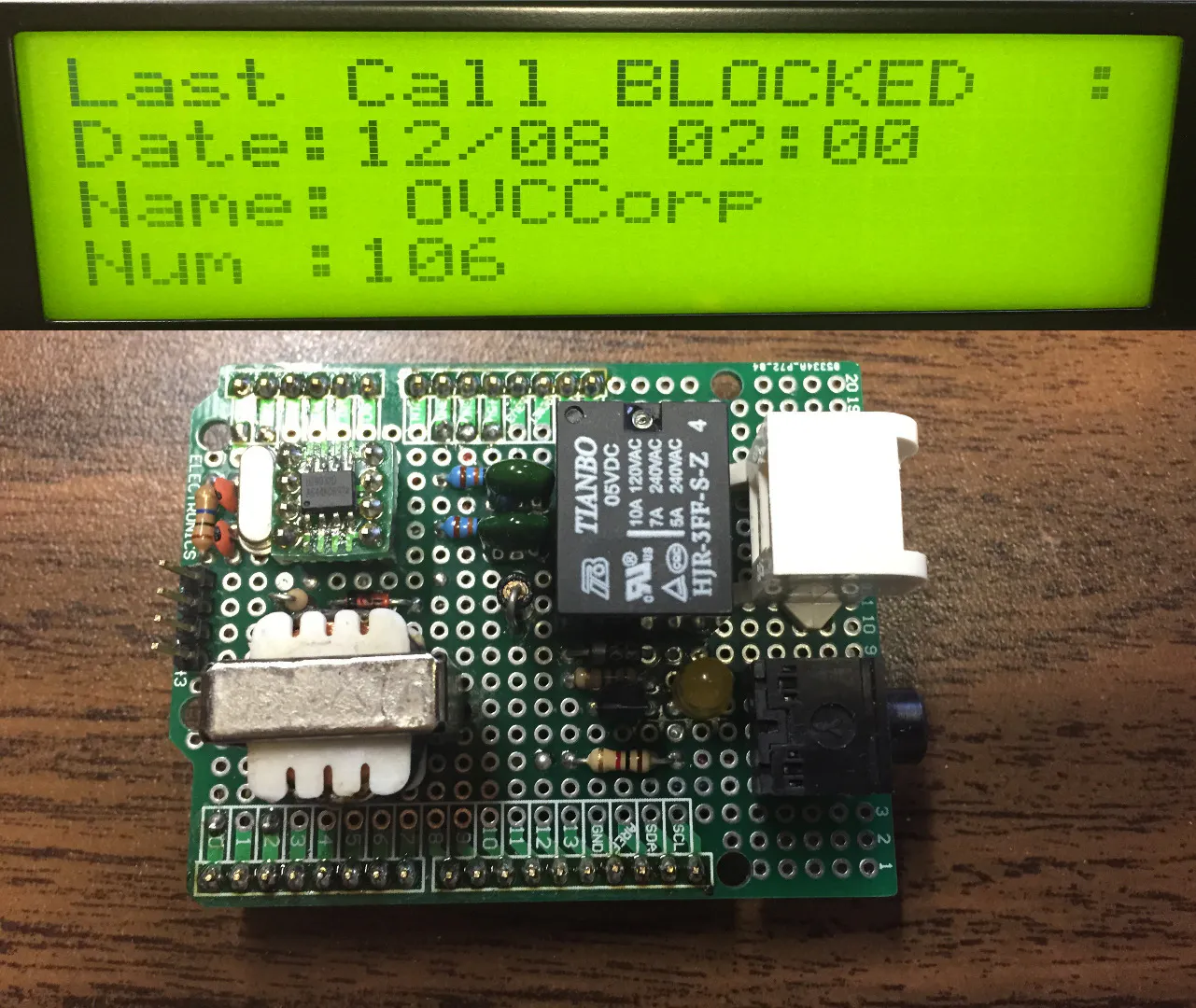Things used in this project

Hardware components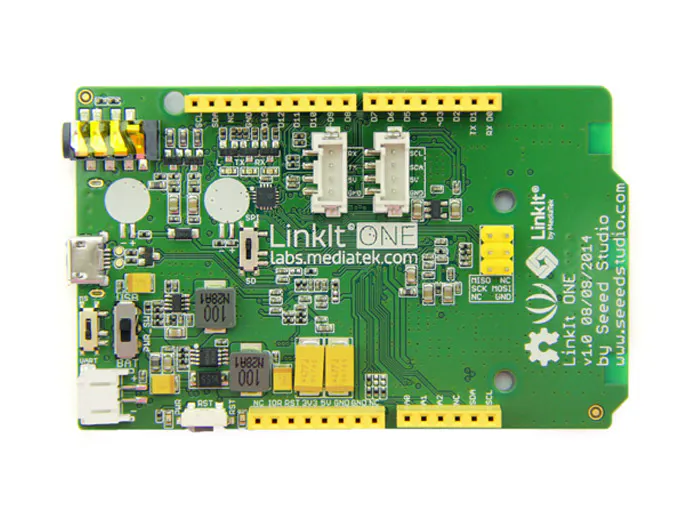×1
 Relay (generic)
×1
 transformer
×1
 3.3V Zener Diode
×3
 ksp013 darlington
×1
×1
 RJ11 Jack
×1
 22pf Capacitors
×2
 200K resistors
×2
 .1uF caps
×2
 HT9032D Caller ID Decoder
×1
×1
 3.58Mhz Crystal
×1
 10Meg Resistor
×1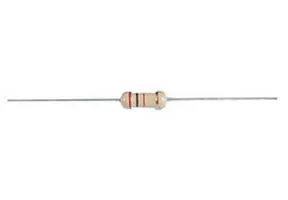Resistor 220 ohm
×1Resistor 1k ohm
×1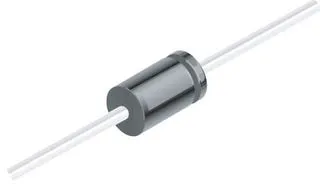1N4007 – High Voltage, High Current Rated Diode
×1

Software apps and online servicesArduino IDE

Schematics

Schematic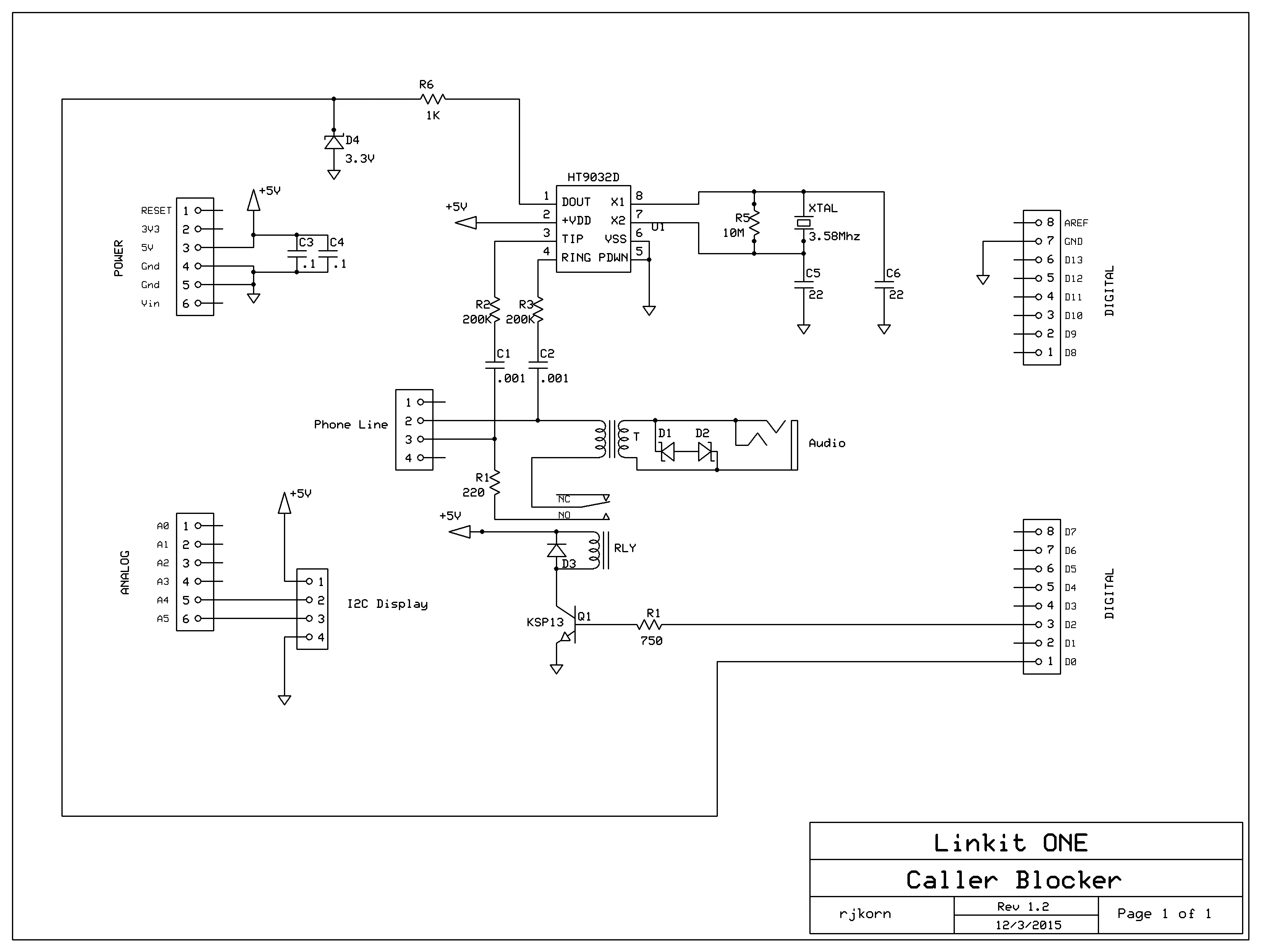Code

CallBlocker.ino

Arduino
For the Arduino IDE
```//****************************************************************
//*  Name    : Caller Blocker                                    *
//*  Author  : Robert Joseph Korn                                *
//*  Date    : 10/18/15                                          *
//*  Version : 1.6                                               *
//*  Notes   :                                                   *
//*          :                                                   *
//****************************************************************

#define Serial_Debug false

#include <Wire.h>
#include <LiquidCrystal_I2C.h>
#include <LAudio.h>
#define HOOK 2

LiquidCrystal_I2C lcd(0x20, 20, 4);

static int state=0;
static int o=0;
static int i=0;
static char nam;
static char num;
static char dat;
static int plen=0;
static int nlen=0;
static int dlen=0;
static int mlen=0;
static int len=0;
int SER = 0;
String str = "";

void BlockCallers() {
if (str == "106"){
lcd.setCursor(0,0);
lcd.print("Last Call BLOCKED  :");
Play_MP3();
}
}

void setup()
{

if(Serial_Debug){
Serial.begin(115200);
while(!Serial){
delay(100);
}
}

pinMode(HOOK, OUTPUT);
digitalWrite(HOOK, LOW);

LAudio.begin();
LAudio.setVolume(6);

lcd.init();
lcd.noBacklight();
delay(400);
lcd.clear();
lcd.backlight();
lcd.setCursor(0,1);
lcd.print("   Caller ID V1.6 ");
lcd.setCursor(0,2);
lcd.print(" Waiting for Caller");
Serial.println("Waiting for next Caller");
delay(3500);

Serial1.begin(1200);
}

void loop()
{
Ht9032_get();

if( state == 10 )
{
o=0;
Serial.println("State: 10 ");
Serial.print("DATE: ");
Serial.print(char(dat));
Serial.print(char(dat));
Serial.print('/');
Serial.print(char(dat));
Serial.println(char(dat));
Serial.print("TIME: ");
Serial.print(char(dat));
Serial.print(char(dat));
Serial.print(':');
Serial.print(char(dat));
Serial.println(char(dat));
Serial.print("NAME: ");
for(int i=0;i<nlen;i++)
{
Serial.print(char(nam[i]));
}
Serial.println("");
Serial.print("NUMBER: ");
for(int i=0;i<mlen;i++)
{
Serial.print(char(num[i]));
}
Serial.println("");

state=0;

lcd.clear();
lcd.setCursor(0,0);
lcd.setCursor(0,1);
lcd.print("Date:");
lcd.print(char(dat));
lcd.print(char(dat));
lcd.print('/');
lcd.print(char(dat));
lcd.print(char(dat));
lcd.print(" ");
lcd.print(char(dat));
lcd.print(char(dat));
lcd.print(':');
lcd.print(char(dat));
lcd.print(char(dat));

lcd.setCursor(0,2);
lcd.print("Name: ");

for(int i=0;i<nlen;i++)
{
lcd.print(char(nam[i]));
}

lcd.setCursor(0,3);
lcd.print("Num :");

str = "";

for(int i=0;i<mlen;i++)
{
lcd.print(char(num[i]));
str += char(num[i]);
}

BlockCallers();
}
}

void Play_MP3()
{
Serial.println("Blocked Caller Detected");
digitalWrite(HOOK, HIGH);
Serial.println("Off Hook");
delay(1500);
Serial.println("Playing message to caller");
LAudio.playFile( storageFlash,(char*)"Klaxon.mp3");
delay(7500);
digitalWrite(HOOK, LOW);
Serial.println("On Hook");
delay(5000);
}

void Ht9032_get()
{
if (Serial1.available() > 0)
{

/*
* states
* 0 - waiting for U's
* 1 - waiting for 25 U's and then an 0x80
* 2 - waiting for packet len
* 3 - waiting for message id
* 4 - get name len
* 5 - receiving name
* 6 - get number len
* 7 - receiving number
* 8 - get date len
* 9 - receiving date
* 10 - packet complete
*
* message ids
* 1 - date
* 2 - number
* 7 - name
*
* reasons
* O - Out of area (no caller ID available)
* P - Private party (Blocked)
*
*/
switch(state)
{
case 0:if (SER==0x55)
{
state=1;
i=1;
Serial.println("State: 0 ");
}break;

case 1:if (SER==0x55)
{
i++;
}

if(SER==0x80)
{
if(i > 25)
{
state=2;
}
}
Serial.println("State: 1 ");
break;

case 2:plen = SER;
i=0;
state=3;
Serial.println("State: 2 ");
Serial.print("Packet Length: ");
Serial.println(plen);
break;

case 3: o++;
Serial.println("State: 3 ");
Serial.print("MID: ");
Serial.println(SER);
if(SER==1)
{
state=8;
}
else if(SER==2)
{
state=6;
}
else if(SER==4)
{
state=6;
}
else if(SER==7)
{
state=4;
}
else if(SER==8)
{
state=4;
}
break;

case 4:len = SER;
nlen = len ;
o++;
i=0;
state=5;
Serial.println("State: 4 ");
Serial.print("Data Length: ");
Serial.println(nlen);
break;

case 6:len = SER;
mlen = len ;
o++;
i=0;
state=7;
Serial.println("State: 6 ");
Serial.print("Data Length: ");
Serial.println(mlen);
break;

case 8:len = SER;
dlen = len ;
o++;
i=0;
state=9;
Serial.println("State: 8 ");
Serial.print("Data Length: ");
Serial.println(dlen);
break;

case 5:nam[i]=SER;
i++;
o++;
if( i > nlen - 1 )
{
state=3;
i=0;
}

if( o > plen - 1 )
{
state=10;
}
Serial.println("State: 5 ");
break;

case 7:num[i]=SER;
i++;
o++;
if( i > mlen - 1 )
{
state=3;
i=0;
}

if( o > plen - 1 )
{
state=10;
}
Serial.println("State: 7 ");
break;

case 9:dat[i]=SER;
i++;
o++;
if( i > dlen - 1 )
{
state=3;
i=0;
}

if( o > plen - 1 )
{
state=10;
}
Serial.println("State: 9 ");
break;

default:break;
}
}
}
```

Credits

Robert Korn

7 projects • 19 followers
I Made the Internet of Things before people had a name for it.